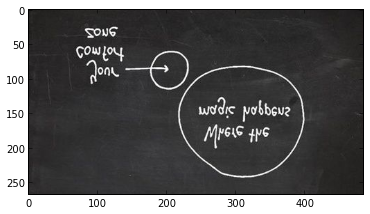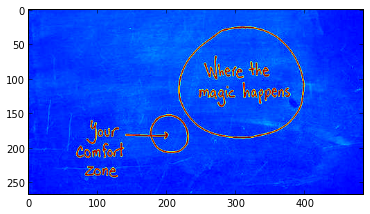In :
import matplotlib.image as mpimg
import urllib2 as urllib
import io
fd = urllib.urlopen("http://mindey.com/wamh.jpg")

In :
imgplot = plt.imshow(img)In :
lum_img = img[:,:,0][::-1]
imgplot = plt.imshow(lum_img)In :
# Let's send it to R
ima = img[:,:,0].astype(numpy.float32)
%Rpush ima

In :
# Let's use R to plot it :)
%R image(t(ima))In :